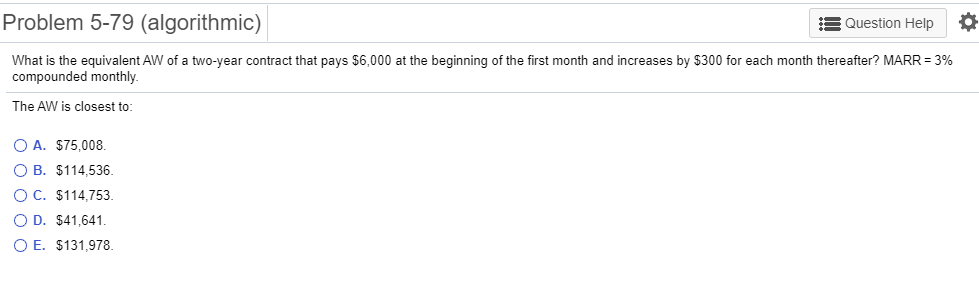### Create an Account

Home / Questions / Problem 5-79 algorithmic Question Help What is the equivalent AW of a two-year

# Problem 5-79 algorithmic Question Help What is the equivalent AW of a two-yearWhat is the equivalent AW of a two​-year contract that pays​\$6,000 at the beginning of the first month and increases by ​\$300 for each month​ thereafter?

MARR= 3​% compounded monthly.

The AW is closest​ to:

Problem 5-79 (algorithmic) Question Help What is the equivalent AW of a two-year contract that pays \$6,000 at the beginning of the first month and increases by \$300 for each month thereafter? MARR = 3% compounded monthly The AW is closest to: O A. \$75,008 O B. \$114,536. O C. \$114,753 O D. \$41,641 O E. \$131,978.

Feb 06 2020 View more View LessGet Solution